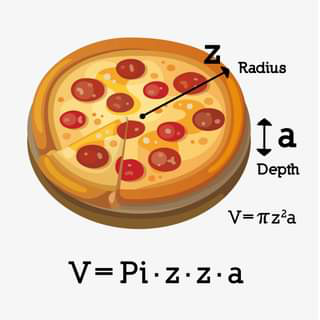A mathematician is a machine for turning coffee into theorems - Alfréd Rényi

## ClassiC DefinitionS and CooL MeaningS

• Lecture: An art of transferring information from the notes of the lecturer to the notes of the students without passing through "the minds of either".
• Conference: The confusion of one man multiplied by the number present.
• Conference Room: A place where everybody talks, nobody listens & everybody disagrees later on.
• Classic: A book which people praise, but do not read.
• Yawn: The only time some married men ever get to open their mouth.
• Boss: Someone who is early when you are late and late when you are early.

## MatH JokeS

• Q: How does one insult a mathematician?
A: You say: "Your brain is smaller than any $\epsilon>0$"
• Q: What does a mathematician present to his fiance when he wants to propose?
A: A polynomial ring!
• A mathematician is asked by a friend who is a devout Christian: "Do you believe in one God?" He answers: "Yes - up to isomorphism."
• The shortest math joke: let epsilon be less than $0$.
• A physicist and an engineer are in a hot-air balloon. Soon, they find themselves lost in a canyon somewhere. They yell out for help: "Helllloooooo! Where are we?" 15 minutes later, they hear an echoing voice: "Helllloooooo! You're in a hot-air balloon!!" The physicist says, "That must have been a mathematician." The engineer asks, "Why do you say that?" The physicist replied: "The answer was absolutely correct, and it was utterly useless."
• Teacher: Now suppose the number of sheep is $x$...
Student: Yes sir, but what happens if the number of sheep is not $x$?
• Take \begin{align*} Radius&=z\\ Width&=a. \end{align*} Then $$Volume=pi\cdot z\cdot\cdot z \cdot a=pizza.$$• Expand $(a+b)^n$.